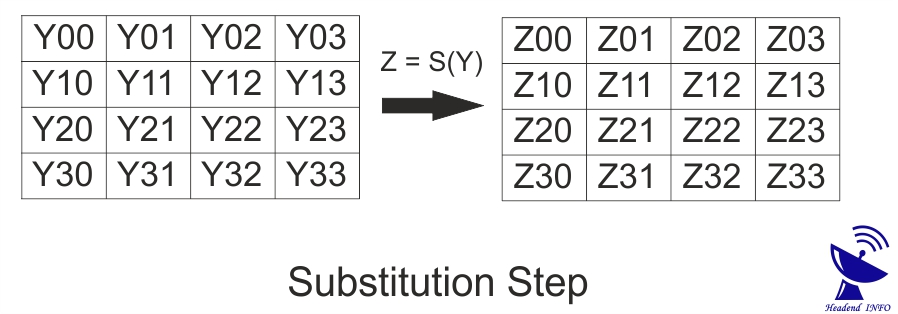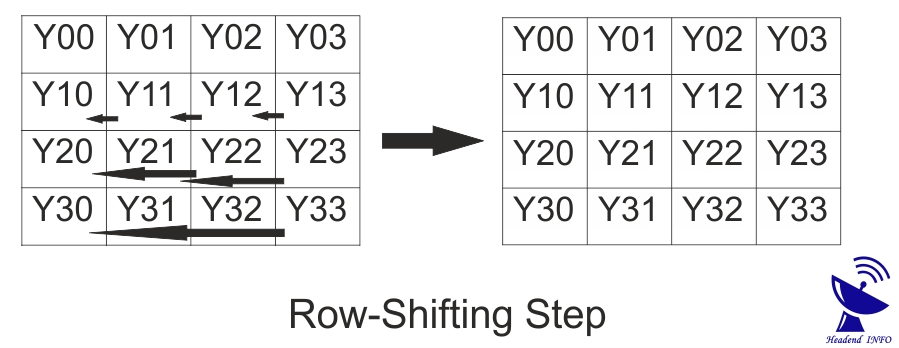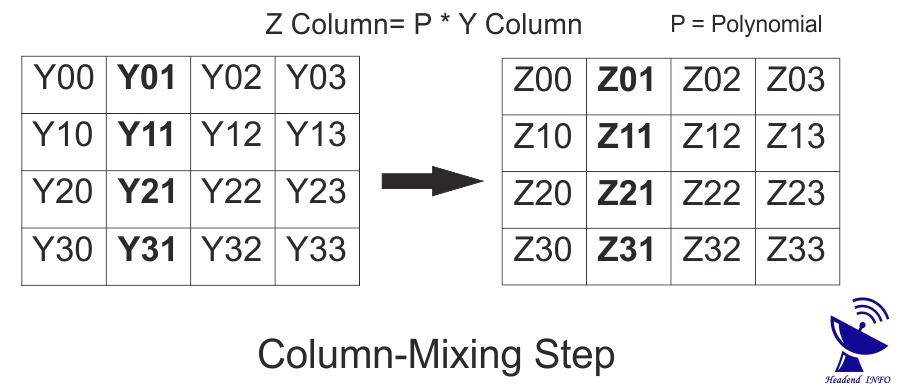# What Is AES Encryption Algorithm | Working Of AES Encryption Algorithm## Introduction Of AES Encryption Algorithm

The AES Encryption Algorithm was approved in year 2000 and then it was published by NIST. NIST is National Institute Of Standards & Technology.

Although various algorithms was submitted by companies but Rijndael was selected. It was submitted by two cryptographers they were from Belgium named as Joan Daemen & Vincent Rijmen.

In 2003 the United States Govt. said we can use AES for security of content. After that it was also approved National Security Agency.

## What Is AES Encryption AlgorithmAES Encryption Algorithm is successor of DES and 3DES. DES is called Data Encryption Standard. There were the need of good encryption algorithm because DES was having small encryption key. The computing power were increasing with time so DES Key became vulnerable.

Although 3DES algorithm removed this problem but it takes lots of time in encryption process. So there were need for a algorithm having long encryption key with faster Encryption and Decryption process which results AES Algorithm.

AES Algorithm is a symmetric key algorithm used to encrypt the premium content from unauthorized users.  AES Algorithm is a symmetric key algorithm so it uses the same KEY for encryption and decryption process.## Working Of AES AlgorithmAs we discussed above AES Encryption Algorithm is a Symmetric Algorithm Cryptography means same Encryption key is used for Encryption Process and for Decryption Process. Before sending the content the receiver should have Encryption Key which receiver can have from a secure and reliable medium.

Where Plaintext is Unencrypted Content which can be accessed by anyone. While Ciphertext represents Encrypted Content for privacy.

The AES Algorithm is made of 3 Block Ciphers AES-128, AES-192 and AES-256. These Block Ciphers are used to encrypt the Data Block of 128 bit.

AES-128 Block CIpher uses the 128-bits Encryption Key Which have 10 Rounds

AES-192 Block CIpher uses the 192-bits Encryption Key Which have 12 Rounds

AES-256 Block CIpher uses the 256-bits Encryption Key Which have 14 Rounds

Where number of round indicates the processing steps followed in encryption to convert a Plaintext into CIphertext.

The processing steps are known as Transformation. The number of transformations depends upon which encryption you are using like 256-bit Encryption Key have 14 Round.

The first step which cipher follow is to adjust the Plaintext into an Array. Then these processing steps are followed,

### (1) Substitution StepIn this step each byte in the array is replaced with its SubByte. For example Y12 replaced with Z12. Where

Z12 = S (Y12) = SubByte of Y12 in Array

Y12 = A Array Byte

Z12 = A Array Byte

S = Substitution Of Byte = SubByte

### (2) Row-Shifting StepIn this step the first row remains same. While the second row is shifted from Right to Left one step. Also these number of shifting increases by one for every next Row.

Y represents a Array Byte.

### (3) Column-Mixing StepIn this step we need to select the one by one column. Where we multiply each column by Polynomial. This Polynomial can be called as “P”.

### (4) Round-Key Addition StepIn this step a round key is added in each byte of array. For addition of round key XOR gate is used as shown in the figure above.

Y = A Array Byte

Z = A Array Byte

R = Round Key

### Conclusion Of AES Encryption Algorithm

AES Encryption Algorithm is a very strong algorithm to secure the content from unauthorized access. It works on 128 -bits fixed data block for encryption. Although Rijndael suggessted that we can increase the 128-bit Data Block for this encryption algorithm with increase in encryption key as well. But it was not adopted. So AES Algorithm was accepted to apply on fixed Data Block of 128-bits.

It is successor of DES and 3 DES in terms of security and speed. Because AES is SIX times faster than 3 DES.

### Know More About   What Is Cryptography

reference : rijndael